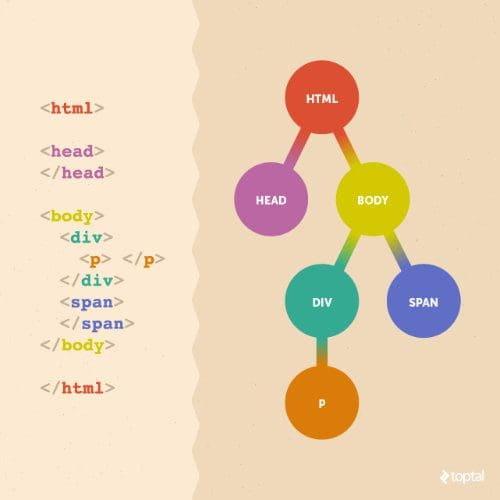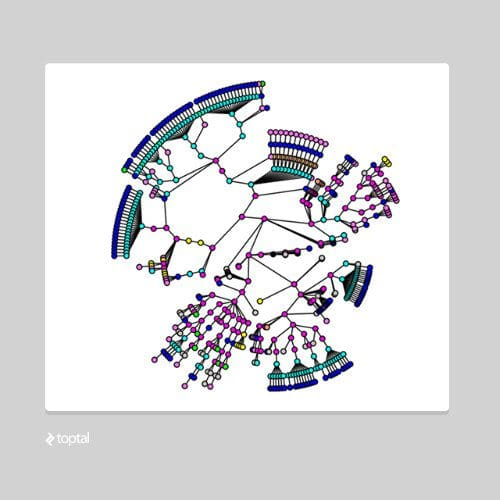# Tree Kernels: Quantifying Similarity Among Tree-Structured Data

An in-depth, informative overview of tree kernels, both theoretical and practical. Includes a use case and some code after the discussion.

Tree Kernel Based on Subpaths

The tree kernel above used a horizontal, or breadth-first, approach to converting trees to strings. While this approach is quite simple, this conversion means it cannot operate on the trees directly in their original form.

This section will define a tree kernel that operates on the vertical structures in trees, allowing the kernel to operate on the tree directly.

A subsection of a path from the root to one of the leaves is called a subpath, and a subpath set is the set of all subpaths included in the tree:Let us assume that we want to define a tree kernel K(T1, T2) between two trees T1 and T2. By using the subpath set, we can define this tree kernel as:Where nump(T) is the number of of times subpath p occurs in tree T, |p| is the number of nodes in subpath p, and P is the set of all subpaths in T1 and T2. w|p| is the weight, similar to that introduced in the previous section.

Here, we present a simple implementation of this kernel using a depth-first search. Although this algorithm runs in quadratic time, more efficient algorithms exist using suffix trees or suffix arrays, or an extension of the multikey quicksort algorithm, which can achieve linearithmic time ( O(|T1|log|T2|) ) on average:

```subpath_track = {}

def generate_subpaths(path, l):
if l == len(path):
if tuple(path) not in subpath_track:
subpath_track[tuple(path)] = 1
else:
subpath_track[tuple(path)] += 1
else:
index = 0
while l+index-1 < len(path):
if tuple(path[index: l+index]) not in subpath_track:
subpath_track[tuple(path[index: l+index])] = 1
else:
subpath_track[tuple(path[index: l+index])] += 1
index += 1

generate_subpaths(path, l+1)

def get_subpaths(graph, root, track, path):
track[root] = True

if graph.degree(root) == 1:
generate_subpaths(path, 1)
else:
for node in graph.neighbors(root):
if node not in track:
get_subpaths(graph, node, track, path + [node, ])

def kernel_subpath(t1, t2, common_track):
kernel_v = 0
for p in subpath_track:
kernel_v += common_track[t1][p]*common_track[t2][p]

return kernel_v
```

In this example, we used the weighting parameter w|s| w|p| = 1. This gives all subpaths equal weighting. However, there are many cases when using k-spectrum weighting, or some dynamically assigned weight value, is appropriate.

Mining Websites

Before we wrap up, let’s briefly look at one real-world application of tree classification: categorization of websites. In many data-mining contexts, it is beneficial to know what “type” of website some data comes from. It turns out that pages from different websites can be categorized quite effectively using trees due to similarities in how web pages for similar services are structured.

How do we do that? HTML documents have a logical nested structure, which is very much like a tree. Each document contains one root element, with additional elements nested inside. Nested elements in an HTML tag are logically equivalent to child nodes of that tag:Let’s take a look at some code that can convert an HTML document into a tree:

```def html_to_dom_tree(root):
node_id = 1
q = deque()
graph = nx.Graph()

q.appendleft({'element': root, "root_id": node_id})
while len(q):
node = q.pop()

if node and node['element'].name == "body":
node_id += 1

root_id = node['root_id']
labels[root_id] = node['element'].name

for t in node['element'].contents:
if t and t.name:
q.appendleft({"element": t, "root_id": node_id})
node_id += 1

return graph
```

This will produce a tree data structure that might look something like this:The implementation above makes use of a couple of helpful Python libraries: NetworkX, for working with complex graph structures, and Beautiful Soup, for pulling data from the web and manipulating documents.

Calling `html_to_dom_tree(soup.find("body"))` will return a NetworkX graph object rooted at the element of the webpage.

Say we want to find groups in a set of 1,000 website homepages. By converting each homepage into a tree like this, we can compare each, for example by using the subpath tree kernel given in the previous section. With these measurements of similarity, we could find that, for example, e-commerce sites, news sites, blogs, and educational sites are easily identified by their similarity to each other.

Conclusion

In this article, we introduced methods for comparing tree-structured data elements to each other, and showed how to apply kernel methods to trees to get a quantifiable measurement of their similarity. Kernel methods have proven to be an excellent choice when operating in high-dimensional spaces, a common situation when working with tree structures. These techniques set the stage for further analysis of large sets of trees, using well-studied methods that operate over the kernel matrix.

Tree structures are encountered in many real-word areas like XML and HTML documents, chemical compounds, natural language processing, or certain types of users behavior. As demonstrated in the example of constructing trees from HTML, these techniques empower us to perform meaningful analysis in these domains.

Bio: Dino Causevic has over five years of experience as a software developer. For the past two years, he has worked in Java and related technologies, mostly in implementing big data solutions using NoSQL technologies and in implementing REST services. He also has experience with .NET and PCI DSS security standards and with REST solutions using Python combined with established frameworks.

Original. Reposted with permission.

Related: